GATE  >  Chemistry - (CY) 2018 GATE Paper (Practice Test)

# Chemistry - (CY) 2018 GATE Paper (Practice Test) - GATE

Test Description

## 65 Questions MCQ Test GATE Past Year Papers for Practice (All Branches) - Chemistry - (CY) 2018 GATE Paper (Practice Test)

Chemistry - (CY) 2018 GATE Paper (Practice Test) for GATE 2023 is part of GATE Past Year Papers for Practice (All Branches) preparation. The Chemistry - (CY) 2018 GATE Paper (Practice Test) questions and answers have been prepared according to the GATE exam syllabus.The Chemistry - (CY) 2018 GATE Paper (Practice Test) MCQs are made for GATE 2023 Exam. Find important definitions, questions, notes, meanings, examples, exercises, MCQs and online tests for Chemistry - (CY) 2018 GATE Paper (Practice Test) below.
Solutions of Chemistry - (CY) 2018 GATE Paper (Practice Test) questions in English are available as part of our GATE Past Year Papers for Practice (All Branches) for GATE & Chemistry - (CY) 2018 GATE Paper (Practice Test) solutions in Hindi for GATE Past Year Papers for Practice (All Branches) course. Download more important topics, notes, lectures and mock test series for GATE Exam by signing up for free. Attempt Chemistry - (CY) 2018 GATE Paper (Practice Test) | 65 questions in 180 minutes | Mock test for GATE preparation | Free important questions MCQ to study GATE Past Year Papers for Practice (All Branches) for GATE Exam | Download free PDF with solutions
 1 Crore+ students have signed up on EduRev. Have you?
Chemistry - (CY) 2018 GATE Paper (Practice Test) - Question 1

### “When she fell down the _______, she received many _______ but little help.” The words that best fill the blanks in the above sentence are

Chemistry - (CY) 2018 GATE Paper (Practice Test) - Question 2

### “In spite of being warned repeatedly, he failed to correct his _________ behaviour.” The word that best fills the blank in the above sentence is

Chemistry - (CY) 2018 GATE Paper (Practice Test) - Question 3

### For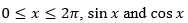are both decreasing functions in the interval ________.

Chemistry - (CY) 2018 GATE Paper (Practice Test) - Question 4

The area of an equilateral triangle is √3. What is the perimeter of the triangle?

Chemistry - (CY) 2018 GATE Paper (Practice Test) - Question 5

Arrange the following three-dimensional objects in the descending order of their volumes:
(i) A cuboid with dimensions 10 cm, 8 cm and 6 cm
(ii) A cube of side 8 cm
(iii) A cylinder with base radius 7 cm and height 7 cm
(iv) A sphere of radius 7 cm

Chemistry - (CY) 2018 GATE Paper (Practice Test) - Question 6

An automobile travels from city A to city B and returns to city A by the same route. The speed of the vehicle during the onward and return journeys were constant at 60 km/h and 90 km/h, respectively. What is the average speed in km/h for the entire journey?

Chemistry - (CY) 2018 GATE Paper (Practice Test) - Question 7

A set of 4 parallel lines intersect with another set of 5 parallel lines. How many parallelograms are formed?

Chemistry - (CY) 2018 GATE Paper (Practice Test) - Question 8

To pass a test, a candidate needs to answer at least 2 out of 3 questions correctly. A total of 6,30,000 candidates appeared for the test. Question A was correctly answered by 3,30,000 candidates. Question B was answered correctly by 2,50,000 candidates. Question C was answered correctly by 2,60,000 candidates. Both questions A and B were answered correctly by 1,00,000 candidates. Both questions B and C were answered correctly by 90,000 candidates. Both questions A and C were answered correctly by 80,000 candidates. If the number of students answering all questions correctly is the same as the number answering none, how many candidates failed to clear the test?

Chemistry - (CY) 2018 GATE Paper (Practice Test) - Question 9

If �� 2 + �� − 1 = 0 what is the value of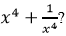Chemistry - (CY) 2018 GATE Paper (Practice Test) - Question 10

In a detailed study of annual crow births in India, it was found that there was relatively no growth during the period 2002 to 2004 and a sudden spike from 2004 to 2005. In another unrelated study, it was found that the revenue from cracker sales in India which remained fairly flat from 2002 to 2004, saw a sudden spike in 2005 before declining again in 2006. The solid line in the graph below refers to annual sale of crackers and the dashed line refers to the annual crow births in India. Choose the most appropriate inference from the above data.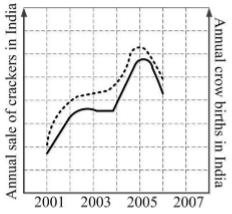Chemistry - (CY) 2018 GATE Paper (Practice Test) - Question 11

The major product formed in the following reaction is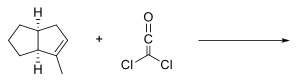Chemistry - (CY) 2018 GATE Paper (Practice Test) - Question 12

The major product formed in the following reaction is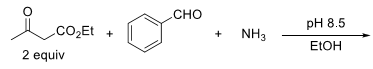Chemistry - (CY) 2018 GATE Paper (Practice Test) - Question 13

The major product of the following intramolecular cycloaddition reaction is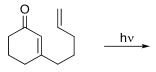Chemistry - (CY) 2018 GATE Paper (Practice Test) - Question 14

The major product of the following reaction is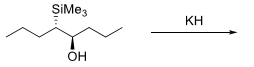Chemistry - (CY) 2018 GATE Paper (Practice Test) - Question 15

The major product formed in the following reaction sequence is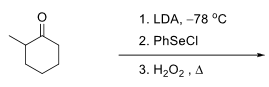Chemistry - (CY) 2018 GATE Paper (Practice Test) - Question 16

The major product formed in the following reaction sequence is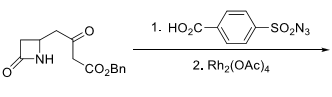Chemistry - (CY) 2018 GATE Paper (Practice Test) - Question 17

The spherical harmonic function,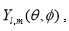with appropriate values of l and m, is an eigenfunction of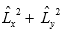operator. The corresponding eigenvalue is

Chemistry - (CY) 2018 GATE Paper (Practice Test) - Question 18

Consider the operators,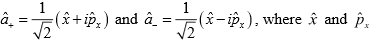are the position and linear momentum operators, respectively. The commutator,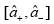is equal to

Chemistry - (CY) 2018 GATE Paper (Practice Test) - Question 19

The temperature derivative of electrochemical cell potential E at constant pressure,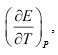is given by

Chemistry - (CY) 2018 GATE Paper (Practice Test) - Question 20

For an ionic micelle-forming surfactant near its critical micelle concentration (CMC), the dependence of molar conductivity and surface tension on surfactant concentration is best represented by

Chemistry - (CY) 2018 GATE Paper (Practice Test) - Question 21

According to Eyring transition state theory for a bimolecular reaction, the activated complex has

Chemistry - (CY) 2018 GATE Paper (Practice Test) - Question 22

Based on Wade’s rule, the structure-type of [B5H8]– is

Chemistry - (CY) 2018 GATE Paper (Practice Test) - Question 23

The coordination geometries around the copper ion of plastocyanin (a blue-copper protein) in oxidized and reduced form, respectively, are

Chemistry - (CY) 2018 GATE Paper (Practice Test) - Question 24

The water exchange rates for the complex ions follow the order

Chemistry - (CY) 2018 GATE Paper (Practice Test) - Question 25

The lowest energy d → d transition of the complexes follow the order

Chemistry - (CY) 2018 GATE Paper (Practice Test) - Question 26

The symmetry label of valence p orbitals of a metal ion in an octahedral ligand field is

Chemistry - (CY) 2018 GATE Paper (Practice Test) - Question 27

The bond angle (Ti-C-C) in the crystal structure ofis severely distorted due to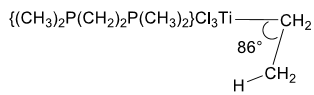Chemistry - (CY) 2018 GATE Paper (Practice Test) - Question 28

The molar heat capacity of a substance is represented in the temperature range 298 K to 400 K by the empirical relation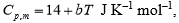where b is a constant. The molar enthalpy change when the substance is heated from 300 K to 350 K is 2 kJ mol-1. The value of b is___________ J K-2 mol-1. (Up to two decimal places)

Chemistry - (CY) 2018 GATE Paper (Practice Test) - Question 29

For low partial pressure of ozone (O3), the adsorption of ozone on graphite surface is fully dissociative in nature, and follows Langmuir isotherm. Under these conditions, if the dependence of the surface coverage of graphite (θ) on partial pressure of ozone ( PO3 ) is given by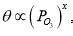the value of x is___________. (Up to two decimal places)

Chemistry - (CY) 2018 GATE Paper (Practice Test) - Question 30

For the radioactive isotope 131I, the time required for 50% disintegration is 8 days. The time required for the 99.9% disintegration of 5.5 g of 131I is ________ days. (Up to one decimal place)

Chemistry - (CY) 2018 GATE Paper (Practice Test) - Question 31

Two moles of an ideal gas X and two moles of an ideal gas Y, initially at the same temperature and pressure, are mixed under isothermal-isobaric condition. The entropy change on mixing is __________J K–1. (Up to one decimal place. Use R = 8.31 J K–1 mol–1)

Chemistry - (CY) 2018 GATE Paper (Practice Test) - Question 32

The energy of a hydrogen molecule in its ground state equilibrium configuration is –31.7 eV. Its dissociation energy is _________ eV. (Up to one decimal place)

Chemistry - (CY) 2018 GATE Paper (Practice Test) - Question 33

The total number of valence electrons in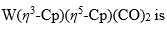______. (Atomic number of W = 74)

Chemistry - (CY) 2018 GATE Paper (Practice Test) - Question 34

In the 1H NMR spectrum of an organic compound recorded on a 300 MHz instrument, a proton resonates as a quartet at d 4.20 ppm. The individual signals of the quartet appear at δ 4.17, 4.19, 4.21 and 4.23 ppm. The coupling constant J in Hz is ______.

Chemistry - (CY) 2018 GATE Paper (Practice Test) - Question 35

In the electron ionization (EI) mass spectra, methyl hexanoate, methyl heptanoate and methyl octanoate give the same base peak. The m/z value of the base peak is ______.

Chemistry - (CY) 2018 GATE Paper (Practice Test) - Question 36

In the following reaction,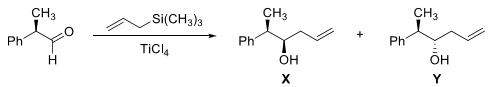Chemistry - (CY) 2018 GATE Paper (Practice Test) - Question 37

The enantiomeric pair, among the following, is

Chemistry - (CY) 2018 GATE Paper (Practice Test) - Question 38

The major product formed in the following reaction sequence is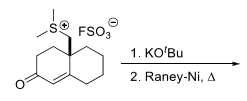Chemistry - (CY) 2018 GATE Paper (Practice Test) - Question 39

The major product in the following reaction sequence is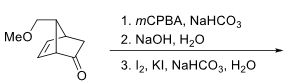Chemistry - (CY) 2018 GATE Paper (Practice Test) - Question 40

The major product formed in the following reaction sequence is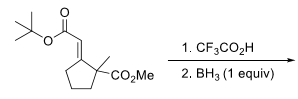Chemistry - (CY) 2018 GATE Paper (Practice Test) - Question 41

The major product of the following reaction sequence is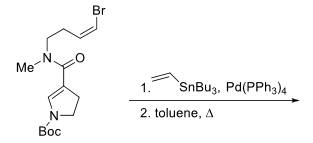Chemistry - (CY) 2018 GATE Paper (Practice Test) - Question 42

The major product formed in the following retro-aldol reaction is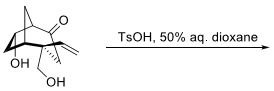Chemistry - (CY) 2018 GATE Paper (Practice Test) - Question 43

The elimination product of the following reaction is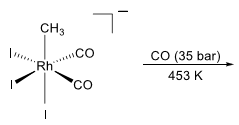Chemistry - (CY) 2018 GATE Paper (Practice Test) - Question 44

A one-dimensional anharmonic oscillator is treated by perturbation theory. The harmonic oscillator is used as the unperturbed system and the perturbation is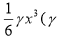is a constant).
Using only the first order correction, the total ground state energy of the anharmonic oscillator is (Note: For a one-dimensional harmonic oscillator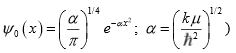Chemistry - (CY) 2018 GATE Paper (Practice Test) - Question 45

The O2 coordinated to metal ion centres in oxy-myoglobin and oxy-hemocyanin exists, respectively, as

Chemistry - (CY) 2018 GATE Paper (Practice Test) - Question 46

Spectroscopic ground state term symbols of cobalt ions in [Co(H2O)6]2+ and [CoCl4]2–, respectively, are

Chemistry - (CY) 2018 GATE Paper (Practice Test) - Question 47

Generally, the coordination number and the nature of the electronic absorption band (f → f transition) of lanthanide(III) ion in their complexes are, respectively,

Chemistry - (CY) 2018 GATE Paper (Practice Test) - Question 48

Second-order rate constant for the reaction between [Co(NH3)5X]n+ (n = 3 for X = NH3 and H2O; n = 2 for X = Cl) and [Cr(H2O)6]2+ at room temperature varies with the X as

Chemistry - (CY) 2018 GATE Paper (Practice Test) - Question 49

For the following reaction sequence,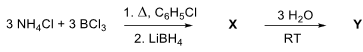X and Y, respectively, are

Chemistry - (CY) 2018 GATE Paper (Practice Test) - Question 50

For an inverse spinel, AB2O4, the A and B, respectively, can be

Chemistry - (CY) 2018 GATE Paper (Practice Test) - Question 51

The reaction of PCl3 with PhLi in 1:3 molar ratio yields X as one of the products, which on further treatment with CH3I gives Y. The reaction of Y with n-BuLi gives product Z. The products X, Y and Z, respectively, are

Chemistry - (CY) 2018 GATE Paper (Practice Test) - Question 52

The reaction of equimolar quantities of Fe(CO)5 and OH gives a complex species X which on further reaction with MnO2 gives species Y. X and Y, respectively, are

Chemistry - (CY) 2018 GATE Paper (Practice Test) - Question 53

The rate constant of a first order reaction,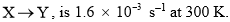Given that the activation energy of the reaction is 28 kJ mol-1 and assuming Arrhenius behavior for the temperature dependence, the total time required to obtain 90% of Y at 350 K is_____________ s. (Up to one decimal place. Use R = 8.31 J K-1 mol-1 )

Chemistry - (CY) 2018 GATE Paper (Practice Test) - Question 54

The molar conductivity of a 0.01 M weak acid (HX) at 298 K, measured in a conductivity cell with cell constant of 0.4 cm–1, is 64.4 S cm2 mol–1. The limiting molar conductivities at infinite dilution of H+ and Xat 298 K are 350 and 410 S cm2 mol–1, respectively. Ignoring activity coefficients, the pKa of HX at 298 K is___________. (Up to two decimal places)

Chemistry - (CY) 2018 GATE Paper (Practice Test) - Question 55

The Latimer diagram of oxygen is given below. The value of x is ________ V. (Up to two decimal places)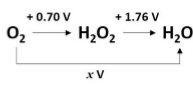Chemistry - (CY) 2018 GATE Paper (Practice Test) - Question 56

At temperature T, the canonical partition function of a harmonic oscillator with fundamental frequency (ν) is given by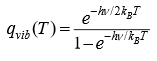For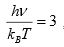the probability of finding the harmonic oscillator in its ground vibrational state is ________. (Up to two decimal places)

Chemistry - (CY) 2018 GATE Paper (Practice Test) - Question 57

The enthalpy of vaporization of a liquid at its boiling point ( Tb = 200 K) is 15.3 kJ mol–1. If the molar volumes of the liquid and the vapour at 200 K are 110 and 12000 cm3 mol–1 respectively, then the slope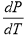of the liquid-vapour boundary is _________ kPa K–1. (Up to two decimal places. Note: 1 Pa = 1 J m–3)

Chemistry - (CY) 2018 GATE Paper (Practice Test) - Question 58

In a molecule XY, let ψ X and ψ Y denote normalized atomic orbitals of atoms X and Y, respectively. A normalized molecular orbital of XY is given by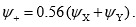. The value of the overlap integral of ψ X and ψ Y is _________________. (Up to two decimal places)

Chemistry - (CY) 2018 GATE Paper (Practice Test) - Question 59

The absorption maxima of two dyes X and Y are 520 and 460 nm, respectively. The absorbance data of these dyes measured in a 1 cm path length cell are given in the table below.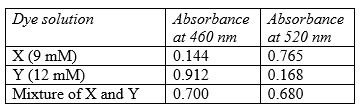The concentration of Y in the mixture is __________ mM. (Up to two decimal places)

Chemistry - (CY) 2018 GATE Paper (Practice Test) - Question 60

The π electrons in benzene can be modelled as particles in a ring that follow Pauli’s exclusion principle. Given that the radius of benzene is 1.4 Å, the longest wavelength of light that is absorbed during an electronic transition in benzene is __________ nm. (Up to one decimal place.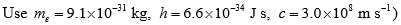Chemistry - (CY) 2018 GATE Paper (Practice Test) - Question 61

The spacing between the two adjacent lines of the microwave spectrum of H35Cl is 6.35 x 1011 Hz . Given that the bond length of D35Cl is 5% greater than that of H35Cl, the corresponding spacing for D35Cl is _______ x 1011 Hz. (Up to two decimal places)

Chemistry - (CY) 2018 GATE Paper (Practice Test) - Question 62

For a diatomic vibrating rotor, in vibrational level u = 3 and rotational level J, the sum of the rotational and vibrational energies is 11493.6 cm–1. Its equilibrium oscillation frequency is 2998.3 cm–1, anharmonicity constant is 0.0124 and rotational constant under rigid rotor approximation is 9.716 cm–1. The value of J is ___________. (Up to nearest integer)

Chemistry - (CY) 2018 GATE Paper (Practice Test) - Question 63

Number of carbonyl groups present in the final product of the following reaction sequence is ___________.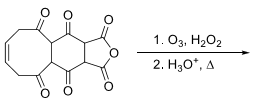Chemistry - (CY) 2018 GATE Paper (Practice Test) - Question 64

A tetrapeptide, made up of natural amino acids, has alanine as the N-terminal residue which is coupled to a chiral amino acid. Upon complete hydrolysis, the tetrapeptide gives glycine, alanine, phenylalanine and leucine. The number of possible sequences of the tetrapeptide is ____.

Chemistry - (CY) 2018 GATE Paper (Practice Test) - Question 65

The strongest band observed in the IR spectrum of the final product of the following reaction appears, approximately, at ______ × 100 cm–1. (Up to one decimal place)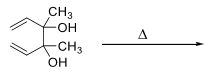## GATE Past Year Papers for Practice (All Branches)

407 docs|127 tests
Information about Chemistry - (CY) 2018 GATE Paper (Practice Test) Page
In this test you can find the Exam questions for Chemistry - (CY) 2018 GATE Paper (Practice Test) solved & explained in the simplest way possible. Besides giving Questions and answers for Chemistry - (CY) 2018 GATE Paper (Practice Test), EduRev gives you an ample number of Online tests for practice

## GATE Past Year Papers for Practice (All Branches)

407 docs|127 tests